Homework Help Question & Answers

# Need some help with SERIES SOLUTION - 2nd ORDER EQUATION For the differential equation,          (1) a. Calculate the indicial equation for the power series solution (Answer in a quadratic polynomial...

Need some help with SERIES SOLUTION - 2nd ORDER EQUATION

For the differential equation,(1)

a. Calculate the indicial equation for the power series solution (Answer in a quadratic polynomial in terms of c.)b. Calculate the solutions of the indicial equation found above.

c. Calculate the pointfrom the above equation (1) as

i. ORDINARY POINT

ii. REGULAR SINGULAR POINT

iii. IRREGULAR SINGULAR POINT

y-Σ@m(z _ 4)nte

#### Homework Answers

Answer #1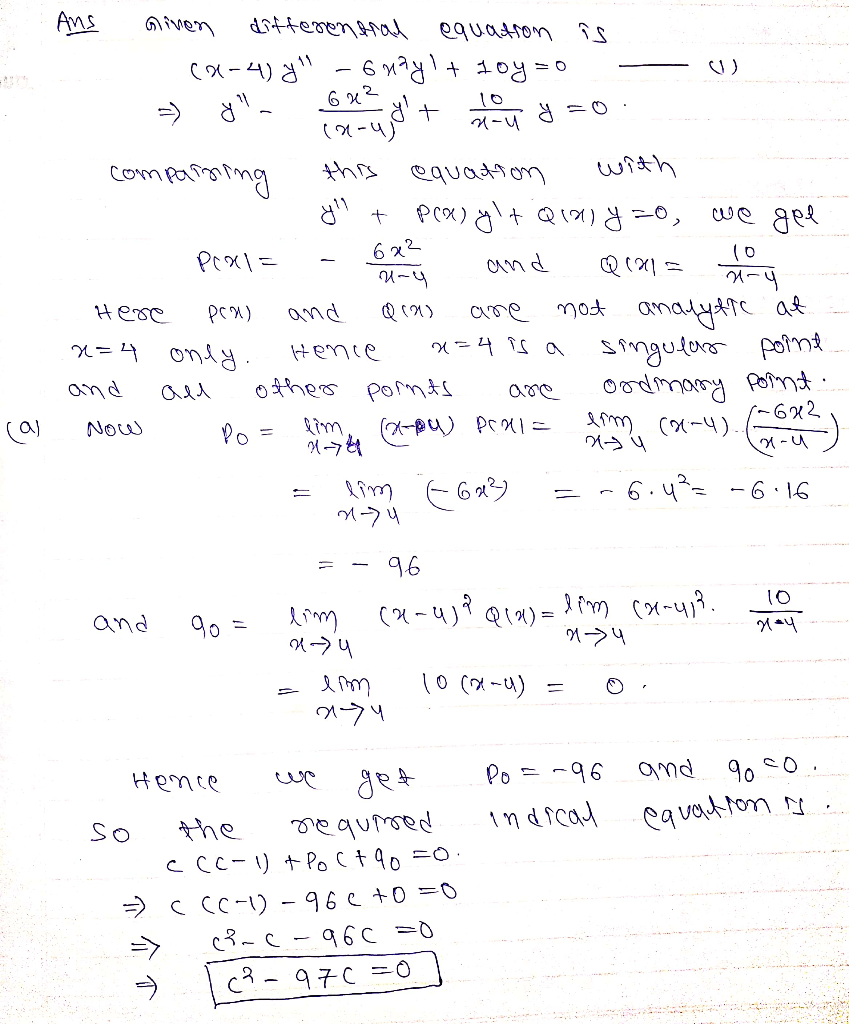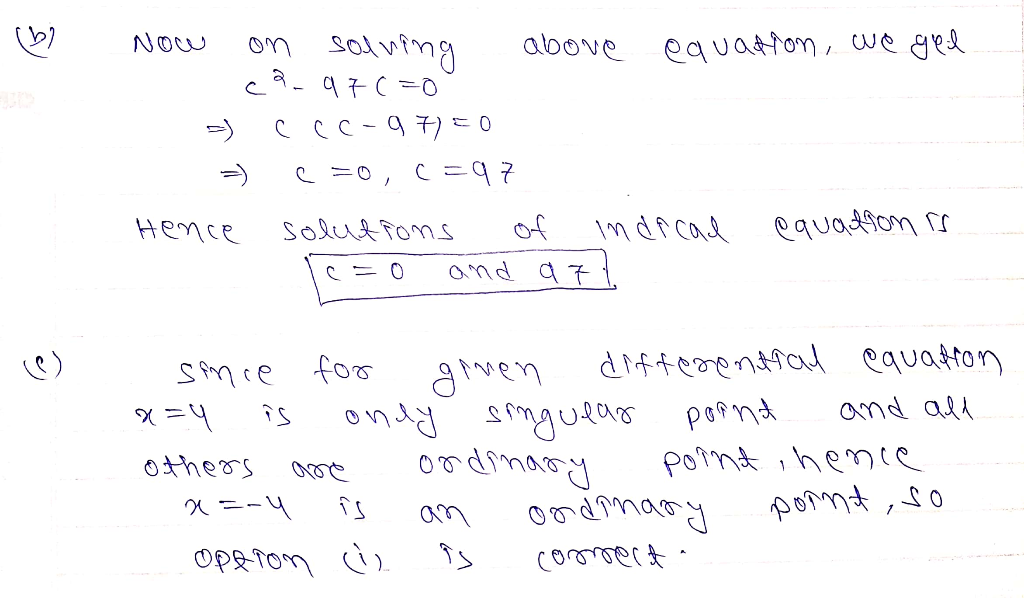Know the answer?
Your Answer:

#### Post as a guest

Your Name:

What's your source?

#### Earn Coin

Coins can be redeemed for fabulous gifts.

Not the answer you're looking for? Ask your own homework help question. Our experts will answer your question WITHIN MINUTES for Free.
Similar Homework Help Questions
• ### (1 point) In this problem you will solve the differential equation or @() (1) Since P(a) 0 are not analytic at and 2()...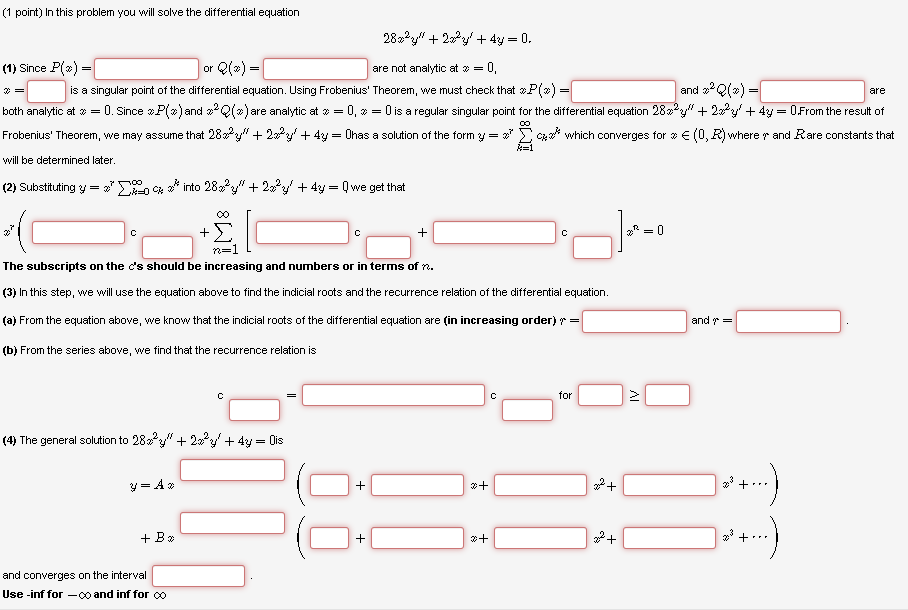(1 point) In this problem you will solve the differential equation or @() (1) Since P(a) 0 are not analytic at and 2() is a singular point of the differential equation. Using Frobenius' Theorem, we must check that are both analytic a # 0. Since #P 2 and #2e(z) are analytic a # 0-0 is a regular singular point for the differential equation 28x2y® + 22,23, + 4y 0 From the result ol Frobenius Theorem, we may assume that 2822y"...

• ### Engineering Mathematics IIA Page 3 of 8 3. Consider the second-order ordinary differential equation for y(x)...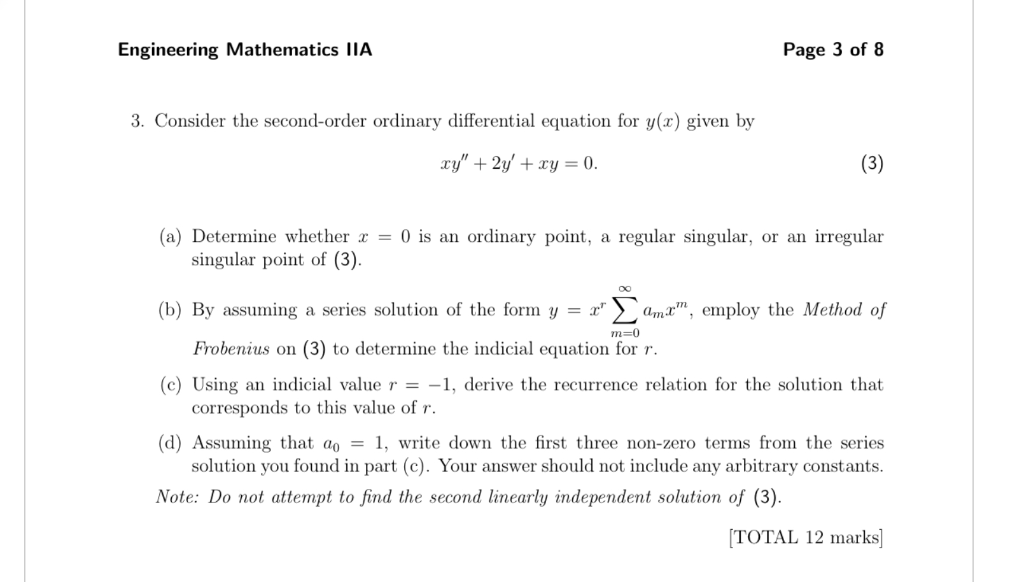Engineering Mathematics IIA Page 3 of 8 3. Consider the second-order ordinary differential equation for y(x) given by (3) xy"2y' +xy = 0. (a) Determine whether = 0 is an ordinary point, regular singular, or an irregular a singular point of (3). (b) By assuming a series solution of the form y = x ama, employ the Method of m-0 Frobenius on (3) to determine the indicial equation for r. (c) Using an indicial value r = -1, derive the...

• ### 7. Consider the differential equation (a) Show that z 0 is a regular singular point of the above differential equation (b) Let y(x) be a solution of the differential equation, where r R and the s...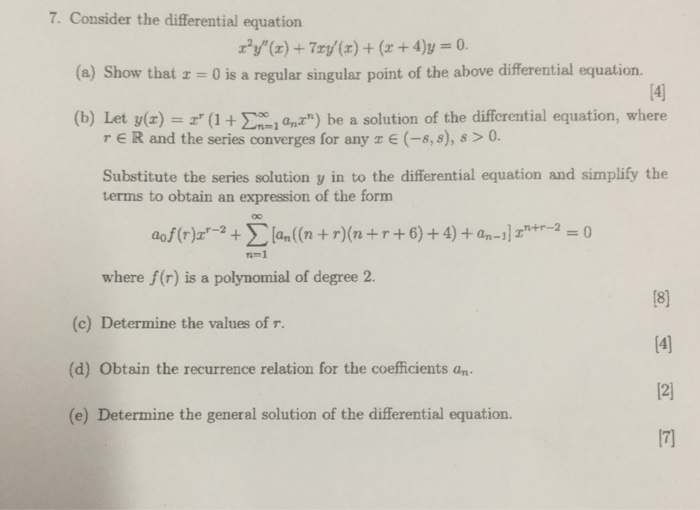7. Consider the differential equation (a) Show that z 0 is a regular singular point of the above differential equation (b) Let y(x) be a solution of the differential equation, where r R and the series converges for any E (-8,s), s > 0 Substitute the series solution y in to the differential equation and simplify the terms to obtain an expression of the form 1-1 where f(r) is a polynomial of degree 2. (c) Determine the values of r....

• ### Consider the following differential equation Note: For each part below you must give your answers in terms of fractions...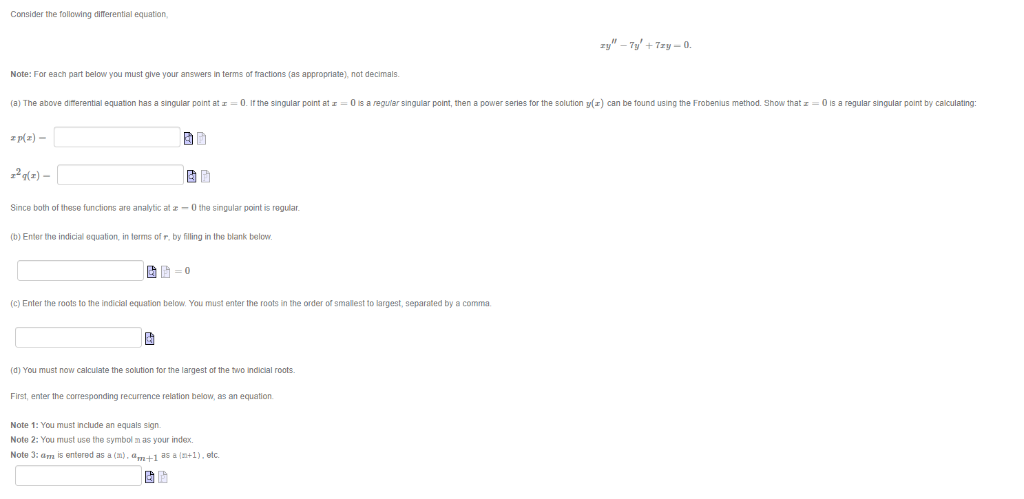Consider the following differential equation Note: For each part below you must give your answers in terms of fractions (as appropriate), not decimals (a) The above difterential equation has a singular point at-0. If the singular point at -0 is a regular singular point, then a power series for the solution y) can be found using the Frobenius method. Show that z-0 is a regular singular point by caliculating p/a)- 2(2) Since both of these functions are analytic at -0...

• ### Consider the following differential equation Note: For each part below you must give your answers in terms of fractions...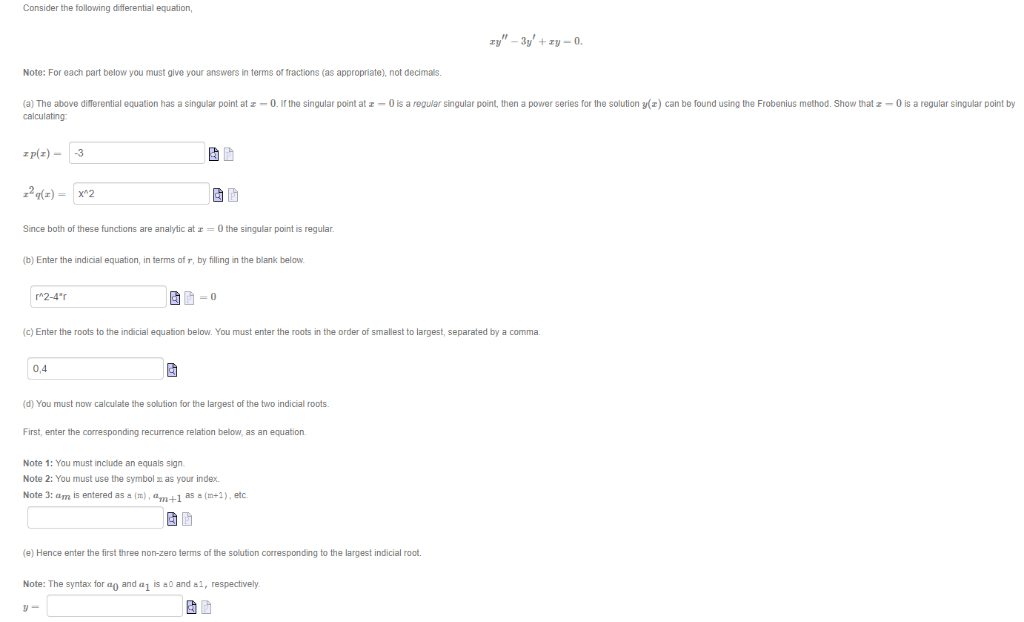Consider the following differential equation Note: For each part below you must give your answers in terms of fractions (as appropriate), not decimas. (a) The above differential equation has a singular point at z-0.I the singular point at z -0 is a regular singular point, then a power series for the solution ()can be found using the Frobenius method. Show that z-O is a regular singular point by calculating plz)-3 Since both of these functions are analytic at r -0...

• ### Solve a 2nd order differential equation with power series solution near a RSP

This is solved near x=0, a regular singular pointx(x-2)y''+2(x-1)y'-2y=0The indicial roots here are equal and I'm having trouble implementing the solution. Please help

• ### (20 pts.) The Laguerre differential equation is ry" + (1 - )y' + Ay = 0....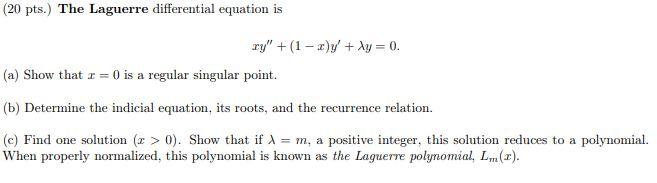(20 pts.) The Laguerre differential equation is ry" + (1 - )y' + Ay = 0. (a) Show that x = 0 is a regular singular point. (b) Determine the indicial equation, its roots, and the recurrence relation. (c) Find one solution (x > 0). Show that if = m, a positive integer, this solution reduces to a polynomial. When properly normalized, this polynomial is known as the Laguerre polynomial, L. (2).

• ### Consider the following differential equation Note: For each part below you must give your answers in terms of fract...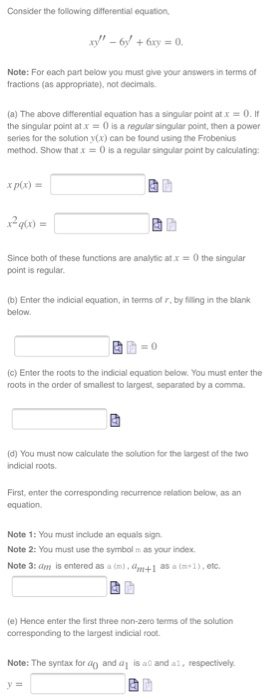Consider the following differential equation Note: For each part below you must give your answers in terms of fractions (as appropriate), not decimals (a) The above differential equation has a snaar point at x 0 . It the singular point at x-0 is a regular singular point, then a power series for the solution y(x) can be lound using the Frobenius method. Show that x = 0 is a regular sigar point by calculating: xp(x) = y(x) = Since both...

• ### 2. Find power series solutions y z" Σ anr" of the following equation centered at 0...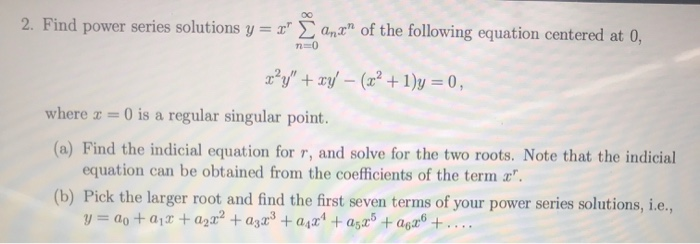2. Find power series solutions y z" Σ anr" of the following equation centered at 0 where-0 is a regular singular point. (a) Find the indicial equation for r, and solve for the two roots. Note that the indicial equation can be obtained from the coefficients of the term Pick the larger root and find the first seven terms of your power series solutions, i.e., (b)

• ### 6: Problem 4 Previous Problem List Next (1 point) Consider the differential equation which has a ...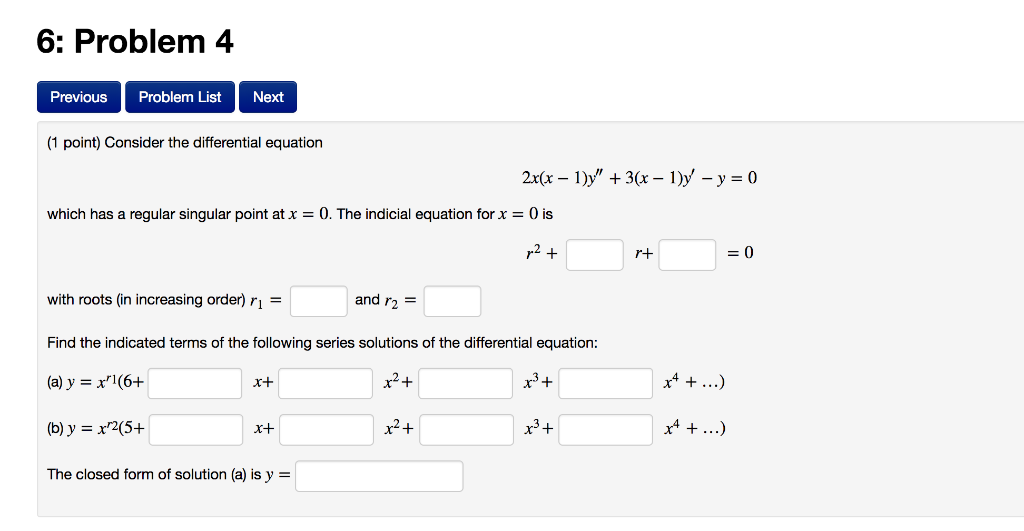6: Problem 4 Previous Problem List Next (1 point) Consider the differential equation which has a regular singular point at x = O. The indicial equation for x = 0 is rt with roots (in increasing order) ri- Find the indicated terms of the following series solutions of the differential equation: (a) y = x,16+ and rE x+ The closed form of solution (a) is y 6: Problem 4 Previous Problem List Next (1 point) Consider the differential equation which...